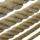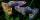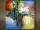# Peppers

In the box are yellow (a), green (b) and red (c) peppers. Their amount is in a ratio 2:4:1 . Most are yellow peppers and green the least. Calculate the number of peppers each type if the total number of peppers is 70.

Result

a =  40
b =  10
c =  20

#### Solution:Leave us a comment of example and its solution (i.e. if it is still somewhat unclear...):

Showing 0 comments:Be the first to comment!## Next similar examples:

1. Arble bagA marble bag sold by Rachel's Marble Company contains 5 orange marbles for every 6 green marbles. If a bag has 35 orange marbles, how many green marbles does it contain?
2. Moneys in triple ratioMilan, John and Lili have a total 344 euros. Their amounts are in the ratio 1:2:5. Determine how much each of them has?
3. AgesJohn, Teresa, Daniel and Paul have summary 56 years. Their ages are in a ratio of 1:2:5:6. Determine how many years have each of them.
4. RunnersFor three runners (on the first to third place) is prepared 30 chocolate that they be distributed in the ratio of 3 : 2 : 1 How much chocolate will get everyone?
5. DonutsFind how many donuts each student will receive if you share 126 donuts in a ratio of 1:5:8
6. StringDivide the string length 800 cm in ratio 1: 5
7. GuppiesAudrey has some guppies in a fish tank. The ration of the oranges guppies to silver guppies is 3:5. She has 12y oranges guppies. Write the number of silver guppies she has in terms of y
8. Image scaleThe actual image dimensions are 60 cm x 80cm and has a reduced size 3 cm x 4 cm. At what scale the image was reduced?
9. Line segmentThe 4 cm long line segment is enlarged in the ratio of 5/2. How many centimeters will measure the new line segment?
10. RatiosReduce the numbers: 50 in a 1:2 ratio 111 at a ratio of 2:3 70 at 10:50 560 at a ratio of 3:8
11. Divide moneyDivide 1200 USD at a ratio of 1:2:3:4:5:6:9:10
12. Land areaA land area of Asia and Africa are in a 3: 2 ratio, the European and African are is 1:3. What are the proportions of Asia, Africa, and Europe?
13. BuildingAt the building, we divided 240 boards into two piles in a 5: 3 ratio. How many were fewer boards in the lower pile?
14. Report 2A School reports students to teacher ratio of 6:1. If there are 45 teachers in the School, how many students are there?
15. One-thirdA one-third of unknown number is equal to five times as great as the difference of the same unknown number and number 28. Determine the unknown number.
16. CakesGrandmother baked cakes. Half of its was poppy, quarter with plum jam and 16 cheesecakes. How many cakes she baked in total?
17. Three digits number 2Find the number of all three-digit positive integers that can be put together from digits 1,2,3,4 and which are subject to the same time has the following conditions: on one positions is one of the numbers 1,3,4, on the place of hundreds 4 or 2.